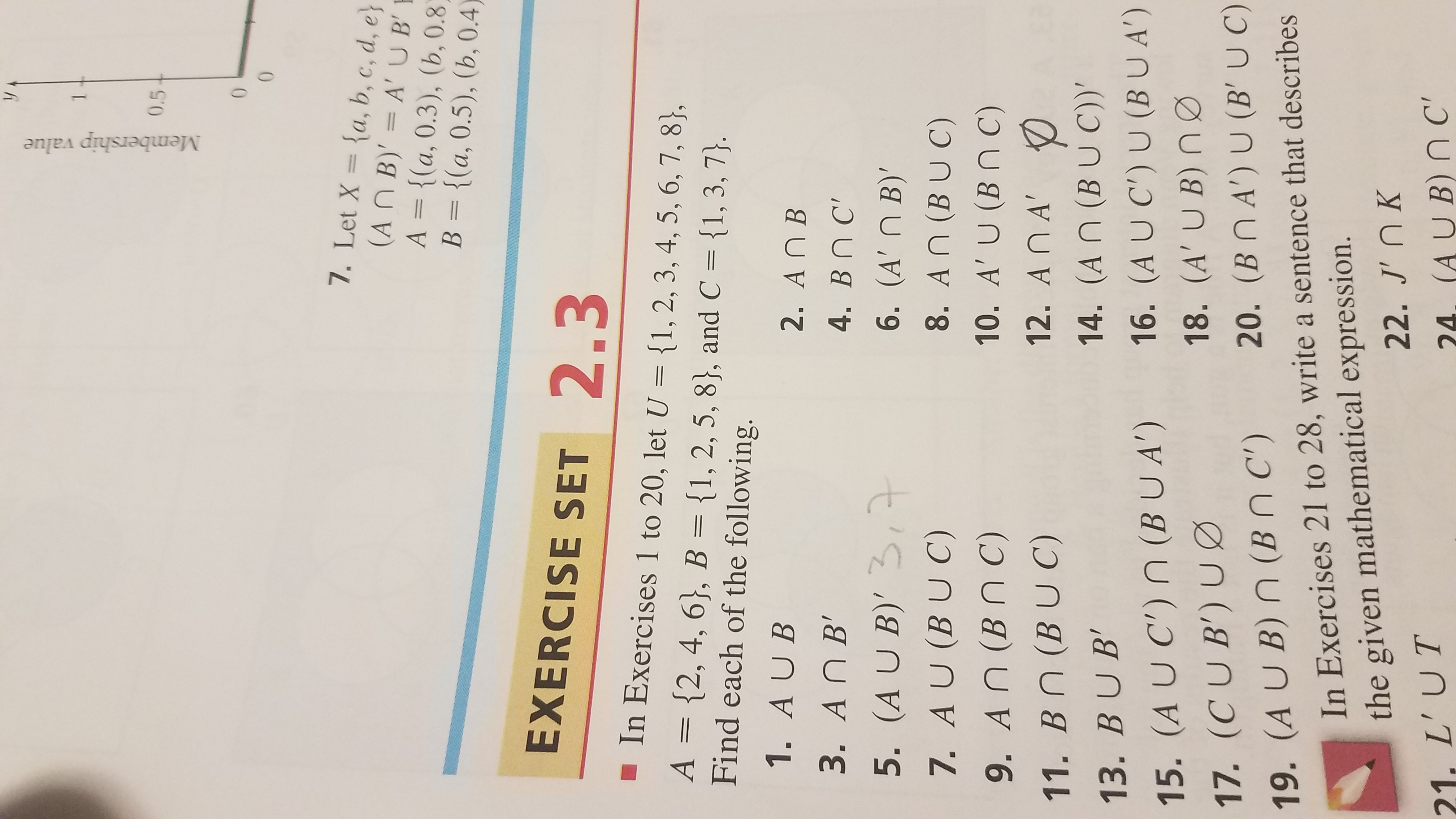# 0.5007. Let X ={a, b, c, d, e(An B)' A'UBA = {(a, 0.3), (b, 0.8)B {(a, 0.5), (b, 0.4)EXERCISE SET 2.3In Exercises 1 to 20, let U = {1, 2, 3, 4, 5, 6, 7, 8},A {2, 4, 6}, B {1, 2, 5, 8}, and C {1, 3, 7}.Find each of the following.-1. AU B2. An B3. An B'4. Bn C'76. (A'n B)'8. An (BUC)5. (A U B)'7. A U (BUC)10. A' U (Bn C)9. An (Bn C)12. An A'11. Bn (BUC)14. (An (BU C))'13. BU B'16. (A UC') U (BUA')15. (A U C') n (BUA')18. (A' U B) n020. (Bn A') U (B' U C)17. (CUB) U19. (A U B) n (Bn C')In Exercises 21 to 28, write a sentence that describesthe given mathematical expression.22. J' nK24, (AU B) n C21. L' UTMembership value

Question

Question 14 and 18 how can solve thanks for your help.help_outlineImage Transcriptionclose0.5 0 0 7. Let X ={a, b, c, d, e (An B)' A'UB A = {(a, 0.3), (b, 0.8) B {(a, 0.5), (b, 0.4) EXERCISE SET 2.3 In Exercises 1 to 20, let U = {1, 2, 3, 4, 5, 6, 7, 8}, A {2, 4, 6}, B {1, 2, 5, 8}, and C {1, 3, 7}. Find each of the following. - 1. AU B 2. An B 3. An B' 4. Bn C' 7 6. (A'n B)' 8. An (BUC) 5. (A U B)' 7. A U (BUC) 10. A' U (Bn C) 9. An (Bn C) 12. An A' 11. Bn (BUC) 14. (An (BU C))' 13. BU B' 16. (A UC') U (BUA') 15. (A U C') n (BUA') 18. (A' U B) n0 20. (Bn A') U (B' U C) 17. (CUB) U 19. (A U B) n (Bn C') In Exercises 21 to 28, write a sentence that describes the given mathematical expression. 22. J' nK 24, (AU B) n C 21. L' UT Membership value fullscreen
check_circleExpert Solution
Step 1

14.

The union of B and C is calculated as follows.

Step 2

The intersection of A and (B C) is calculated as follows.

Step 3

Here, the complement of the set A ꓵ (B C) is the set of elements that are members of the set U but are not in A ꓵ (B &...

### Want to see the full answer?

See Solution

#### Want to see this answer and more?

Solutions are written by subject experts who are available 24/7. Questions are typically answered within 1 hour*

See Solution
*Response times may vary by subject and question
Tagged in

### Math